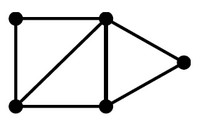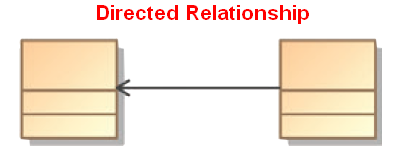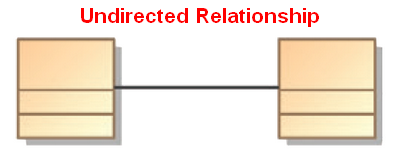# Logical Data Modeling - Relationship Direction (Directed/Undirected)

The direction of a relationship shows if the relationship can be interpreted in one or both directions.

There is only two options:

## Type

### Directed

A directed relationship models asymmetric relations (ie the relationship can be interpreted in only one direction)

It represents a Relationship between a collection of source model elements and a collection of target model elements. A Directed Relationship is said to be directed from the source elements to the target elements.

### Undirected

An Undirected relationship models a symmetric relations (ie the relationship can be interpreted in both directions)

Discover MoreData Modeling - Reference (connect, link to)

Reference is the name of a relationship that is directed where an entity (source, first) refers to another entity (target, second) We say that the source entity references, cites, or otherwise points...Graph - Direction

A direction is a property of an edge. Directed edges are suitable for modeling asymmetric relations Undirected edges are suitable for modeling symmetric relations An edge is directed if it has a...Logical Data Modeling - Antisymmetry relationship

A Antisymmetric relation is a relationship that happens when for all a and b in X: if a is related to b then b isNOT related to a or b=a (reflexivity is allowed) In mathematical notation, an Antisymmetric...Logical Data Modeling - Asymmetric Relation (Uni-directional|Antisymmetric)

An asymmetric relation is a type of binary relation that requiers: antisymmetry (ie if a is related to b, b is not related to a) and irreflexivity (ie an element cannot be related to itself) irreflexivity...Logical Data Modeling - Symmetric relationship (bi-directional)

A symmetric relation is a type of binary relation that happens when for all a and b in X if a is related to b, then b is related to a In mathematical notation, the relation f between x and y is...